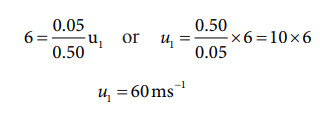Home | | Physics 11th std | Solved Example Problems for Perfect inelastic collision

# Solved Example Problems for Perfect inelastic collision

Physics : Work, Energy and Power : Perfect inelastic collision

Solved Example Problems for Perfect inelastic collision

### Example 4.21

A bullet of mass 50 g is fired from below into a suspended object of mass 450 g. The object rises through a height of 1.8 m with bullet remaining inside the object. Find the speed of the bullet. Take g = 10 ms-2.

### Solution

m1 = 50 g = 0.05 kg; m2 = 450 g = 0.45kgThe speed of the bullet is u1. The second body is at rest u2 = 0 . Let the common velocity of the bullet and the object after the bullet is embedded into the object is v.The combined velocity is the initial velocity for the vertical upward motion of the combined bullet and the object. From second equation of motion,Substituting this in the above equation, the value of u1 isStudy Material, Lecturing Notes, Assignment, Reference, Wiki description explanation, brief detail
11th Physics : UNIT 4 : Work, Energy and Power : Solved Example Problems for Perfect inelastic collision |